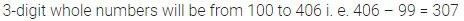# ML Aggarwal Class 6 Solutions for ICSE Maths Chapter 2 Whole Numbers Ex 2.1

## ML Aggarwal Class 6 Solutions for ICSE Maths Chapter 2 Whole Numbers Ex 2.1

Question 1.
Write the smallest whole number. Can you write the largest whole number?
Solution: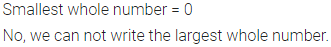Question 2.
Write the successor of each of the following numbers:
(i) 3999
(ii) 378915
(iii) 5001299
Solution: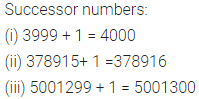Question 3.
Write the predecessor of each of the following numbers:
(i) 500
(ii) 38794
(iii) 54789011
Solution: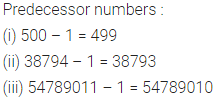Question 4.
Write the whole number (in each of the following) whose successor is :
(i) 50795
(ii) 720300
(iii) 8300000
Solution:Question 5.
Write the whole number (in each of the following) whose predecessor is:
(i) 5347
(ii) 72399
(iii) 3012999
Solution: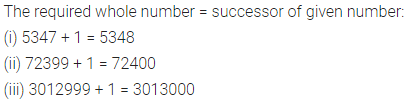Question 6.
Write next three consecutive whole numbers of the following numbers:
(i) 79
(ii) 598
(iii) 35669
Solution:Question 7.
Write three consecutive whole numbers occuring just before 320001.
Solution: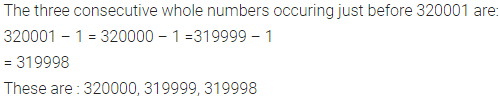Question 8.
(i) How many whole numbers are there between 38 and 68?
(ii) How many whole numbers are there between 99 and 300?
Solution: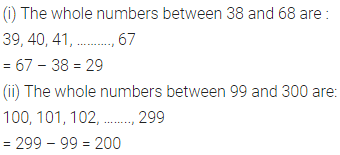Question 9.
Write all whole numbers between 100 and 200 which do not change if the digits are written in reverse order.
Solution: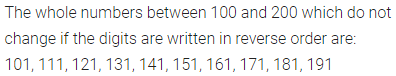Question 10.
How many 2-digit whole numbers are there between 5 and 92?
Solution: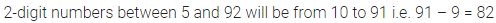Question 11.
How many 3-digit whole numbers are there between 72 and 407?
Solution: